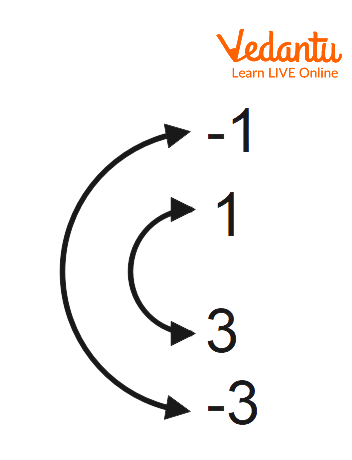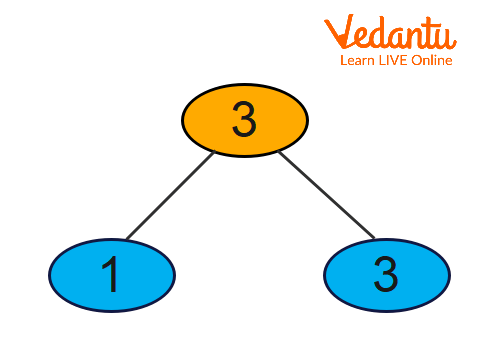Courses
Courses for Kids
Free study material
Offline Centres
More

# Factors of 3Last updated date: 02nd Dec 2023
Total views: 58.2k
Views today: 1.58k## Factors of 3: An Introduction

A factor in mathematics is a divisor of a given number that divides it precisely with no remainder. To determine a number's components, we can adopt several methods, such as the division method and the multiplication approach. Only 3 and 1 are factors of 3. Both positive and negative factors are multiples of three. Both positive and negative integers are possible for the factors and pair factors of 3. For instance, the pair factor of three is represented as (1, 3) or (-1, -3).

The number 3 is the result of multiplying -1 and -3. Using the prime factorization approach and numerous solved cases, we will discover the factors of 3, pair factors of 3, and the prime factors of 3. You will learn about the three factors in this section, and for your benefit, we'll calculate the three factors' sums, identify their prime factors, and display them in pairs.

## What are the Factors of 3?

The numbers that, when multiplied by 3, leave a remainder of zero are considered factors of a 3. Only factors 1 and 3 make up the number 3.

Keep in mind that ${-1 \times (-3)}=3$. As a product of any two negative numbers yields a positive number, (-1, -3) are also factors.

However, let's limit our discussion to positive integers in this lecture.

## How to Calculate Factors of 3?

Let's understand how to calculate the factors of three.

Step 1: Write down the number that needs to be factored in first. The number is 3.

Step 2: Find the two numbers whose product equals 3 in step two.

We get $1 \times 3=3$.

The factors are, therefore 1 and 3.

## Factors of 3 Using Division Method

In the division method, the integer numbers are divided by the number 3 to determine the factors of 3. The number is a factor of 3 if it divides by 3 precisely. Divide the third number by one now, then do the same with the second and third numbers.

$3 \div 1=3$ (Factor is 1 and Remainder is 0)

$3 \div 3=1$ (Factor is 3 and Remainder is 0)

When the number 3 is divided by two, the result is one, hence two is not a factor of three. As a result, 1 and 3 are the factors of 3.

## Prime Factorization of 3

The prime factorization of 3 is expressing the number 3 as the product of its prime factors. To use the prime factorization method to determine the prime factors of 3, follow the steps below.

Take the pair factor of 3, for example (1, 3)

We are unable to divide further since the number 3 in this case is a prime number.

So, 3 is represented as $1 \times 3$.

Consequently, ($1 \times 3$) is the prime factorization of 3.

Note: If a pair factor contains a composite number, divide it into its prime factors and write the resulting numbers as the product of those prime factors.

## Factor Pairs of 3

Factor pairs of three consist of two factors combined so that their output equals three. The positive factor pairs of three are listed below.

$1 \times 3 = 3$

$3 \times 1 = 3$

Negative values are included in factors of 3, as we mentioned earlier. The list of positive factor pairs above can be changed to a list of negative factor pairs of three by simply adding a minus sign in front of each factor:

$-1 \times -3 = 3$

$-3 \times -1 = 3$Pair Factors of 3

Thus, we have positive and negative factors pairs:

(1,3), (3,1), (-1,-3) and (-3,-1).

## Factor Tree

To determine a number's prime factors, a factor tree is constructed. It is constructed as a tree, with the supplied number's branches standing in for each of the number's factors. Let's learn more about the factor tree approach for determining a number's factors. See the factor tree of 3 in the accompanying figure.Factor tree

## Solved Examples

Example 1: To find out if 3 is a prime or a composite number, Bansi looks at the number.

Solution: Let's begin by compiling a list of all the 3's factors.

Factors 1 and 3 are involved.

If a number solely consists of the number itself and one other element, such as 1. This quantity is prime.

A number is considered composite if it has more than two elements.

The number 3 is a prime number since it only has two elements.

Example 2: During a class test, Rojer was asked to find the common factors between 3 and 12. Aid Rojer in figuring out the issue.

Solution: Prime factorization may be used to get the factors of 12:

We get:

12 can be factored into its primes as $2 \times 2 \times 3$

As $1 \times 3$ is the prime factorization of 3,

The common factor between 3 and 12 is 3.

## Practice Problems

1. Shri is calculating the total number of factors that equal 3. Can you locate it for him?

1. 2

2. 1

3. 13

4. 3

Ans: Option A

2. What is the greatest number that 3 and 5 have in common?

1. 5

2. 3

3. 2

4. 1

Ans: Option D

## Summary

Each factor can be combined with another to create the number 3, obtained by multiplying the two. In this instance, 3 is a prime number. When you reduce the factors of 3 to just the prime factors and express 3 as the product of those prime factors, you are said to have performed prime factorisation. The number is a factor of 3 if it divides by 3 precisely.

When the number 3 is divided by two, the result is one; hence two is not a factor of three. As a result, 1 and 3 are the factors of 3. A number's factors, and more specifically, its prime factorization, can be expressed as factor trees. There are factors for each branch of the tree.

## FAQs on Factors of 3

1. How many prime factors are there in 3?

Prime factorization of 3 is $1 \times 3$

Three have factors one and three. We can see that the number 3 only has two elements. As a result, there are only 2 numbers that completely divide 3 without producing a residue.

2. What is a factor in Mathematics?

A number or algebraic expression that evenly divides another number or expression—i.e., leaves no remainder—is referred to as a factor in mathematics. As an illustration, 3 and 6 are factors of 12 because $12 \div 3= 4 and 12 \div 6=2$, respectively. 1, 2, 4, and 12 are the other components that make up 12. A positive integer or algebraic expression with two or fewer factors (i.e., itself and 1) greater than one is referred to as prime; one with more factors is referred to as composite.

3. What variables divide by three?

f a number's total digits equal a multiple of three or is divisible by three, it is said to be three-divisible. Take into account the multiples of 3: 3, 6, 9, 12, 15, 18, 21, 24, 27, 30. The sum of the digits is always divisible by 3. If a number can be divided by three, then its digit sum can also be.